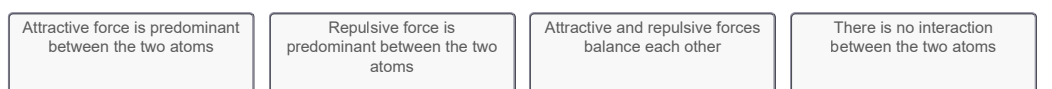# Problem: Part B. Two hydrogen atoms interact to form a hydrogen molecule. Classify the following statements that describe the stages of bond formation in a hydrogen molecule according to the predominant force existing between the two hydrogen atoms.The two hydrogen atoms approach each other to form a bond.The two hydrogen atoms start to combine to form a hydrogen molecule.The internuclear distance between the two hydrogen atoms is less than the bond length.The potential energy of the system is positive.The potential energy of the system is at minimum.The two hydrogen atoms form a stable hydrogen molecule.The two hydrogen atoms are very far apart.Categories: Drag the appropriate items to their respective bins. The changes in the potential energy associated with the formation of a hydrogen molecule from individual hydrogen atoms is represented by the potential energy curve.Initially, when the two hydrogen atoms are far apart, there is no interaction between them. However, as the two atoms come closer together, attraction and repulsion between the two atoms occur. For the formation of a chemical bond to occur, the attractive forces must overcome the repulsive forces. As the attractive force increases, the internuclear distance decreases and the potential energy of the system decreases. At the point where the potential energy is at a minimum, the attractive forces are balanced by the repulsive forces and a stable hydrogen molecule is formed. The internuclear distance corresponding to this point is called the bond length. For hydrogen molecules, bond length is equal to 74 pm.If the internuclear distance between the hydrogen atoms decreases further, then this leads to repulsion between the atoms, thereby increasing the potential energy of the system and creating instability within the molecule.

###### FREE Expert Solution
79% (457 ratings)View Complete Written Solution
###### Problem Details

Part B. Two hydrogen atoms interact to form a hydrogen molecule. Classify the following statements that describe the stages of bond formation in a hydrogen molecule according to the predominant force existing between the two hydrogen atoms.

The two hydrogen atoms approach each other to form a bond.

The two hydrogen atoms start to combine to form a hydrogen molecule.

The internuclear distance between the two hydrogen atoms is less than the bond length.

The potential energy of the system is positive.

The potential energy of the system is at minimum.

The two hydrogen atoms form a stable hydrogen molecule.

The two hydrogen atoms are very far apart.

Categories:Drag the appropriate items to their respective bins.

The changes in the potential energy associated with the formation of a hydrogen molecule from individual hydrogen atoms is represented by the potential energy curve.

Initially, when the two hydrogen atoms are far apart, there is no interaction between them. However, as the two atoms come closer together, attraction and repulsion between the two atoms occur. For the formation of a chemical bond to occur, the attractive forces must overcome the repulsive forces. As the attractive force increases, the internuclear distance decreases and the potential energy of the system decreases. At the point where the potential energy is at a minimum, the attractive forces are balanced by the repulsive forces and a stable hydrogen molecule is formed. The internuclear distance corresponding to this point is called the bond length. For hydrogen molecules, bond length is equal to 74 pm.

If the internuclear distance between the hydrogen atoms decreases further, then this leads to repulsion between the atoms, thereby increasing the potential energy of the system and creating instability within the molecule.